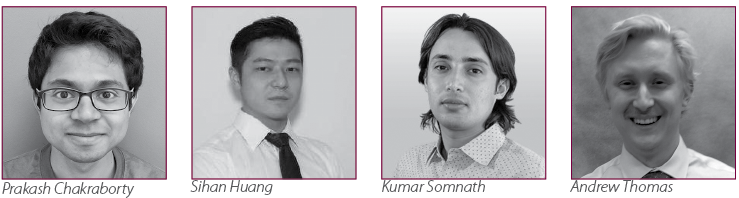Contributing Editor Anirban DasGupta writes the solution to puzzle 24:

Congratulations to the four student members who sent in correct answers—some more complete than others. They are Prakash Chakraborty, Purdue University; Sihan Huang, Columbia University; Kumar Somnath, The Ohio State University; and Andrew Thomas, Purdue University.Now for the solution. Denote the number of steps required to reach the point $(n,n)$ by $S_n$. The quickest that the particle can reach the point $(n,n)$ is in $2n$ steps, which happens if exactly $n$ heads and $n$ tails are produced in $2n$ tosses of our fair coin. This has probability $\frac{{2n \choose n}}{2^{2n}}$.

Next, for any given integer $k \geq 1, P(S_n = 2n+k) = {2n+k-1 \choose n-1}\,2^{-2n-k+1}$.

Thus, $\mu _n = E(S_n) = 2n+\sum_{k=1}^\infty k\,{2n+k-1 \choose n-1}\,2^{-2n-k+1} = 2n\,\bigg (1+\frac{{2n \choose n}}{2^{2n}}\bigg )$, with a little bit of calculation. In particular, $\mu_3 = \frac{63}{8} = 7.875$, and on using Stirling’s series for $n!$, we get
$\mu_n = 2n+\frac{2\,\sqrt{n}}{\sqrt{\pi }}-\frac{1}{4\,\sqrt{n\pi }} +O(n^{-3/2})$.